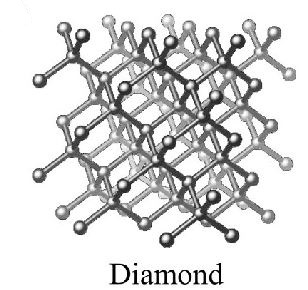# Energy Band in Solids

Energy Band Theory in Solids

Electrons orbit around the nucleus at various levels called shells. An electron which orbit around the nucleus of an atom has potential energy, centrifugal energy, rotational energy and magnetic energy and all of these energies together determine the total energy or energy level of an electron. This energy level of an electron is measured in electron volts which are expressed as ‘eV’. An electron volt is defined as that amount of energy gained or lost by an electron when it moves with or against a potential difference of one volt. In any solid material the atoms are very close to each other and so the outermost valence electrons will interact with one another. But two electrons with opposite spin only can have the same energy level. When more atoms are present new levels are to be established. They are very much nearer to one another but they are separated from one another. This group of related energy level in a polyatomic material is called an energy band. Each energy band consists as many separate levels as there are atoms in the material.

About 1026 atoms are contained in a crystal weighing one kg and the width of the band will normally be 0.1 J (atto Joule = 10-18 Joules = 0.6243 eV) but there will be 14 x 1026 levels spread over the above and width 0.1 aJ. Then the spacing of the level will be less than 10-25 aJ. m specified the number of levels in the individual atoms the value of which is 2 in 'b' band and 6 in 'P' band and so on. The energy levels within a band are therefore discrete but of such small separation that the band can be taken as continuous to the first approximation. Energy band for a solid is shown in Figure.
In the Figure the upper most band is the conduction and in which the electrons are free to move by the application of electric field. An atom having many electrons in the conduction band acts as a good conductor of electricity. Just below the conduction band, there are energy levels forming the forbidden band. In the forbidden band no electrons is found in the natural state but electrons may jump from the valence band to the conduction band or vice versa. It should be noted that electrons cannot remain in the forbidden band. For an insulator the width of the forbidden gap is more and for a conductor it is least. In the case of semiconductor, the forbidden gap is between that of conductor and insulator. See Figure above. A little below this level there is valence band which is formed by series of energy levels. The electrons in these levels are called valence electrons. By the application of energy the electrons from this band may be made to move to conduction band.

The two elements commonly used of the fourth column of the periodic system of elements are Germanium and Silicon. The electron configuration of Germanium and Silicon are shown in Figure below.
In the solid state they crystallize into a diamond structure as shown in Figure (a). The schematic two dimensional representations of the electron pair in the diamond structure are shown in Figure (b). The dots represent electrons. In the actual three dimensional structure the electron pair bands emerge from a given atom to the corners of a rectangular tetrahedron, the angles between the bonds being approximately 109°. The bonds between a given atom in this structure and its neighbors are called electron pair bonds.Figure (a)Figure (b)
Since each atom has four valence electrons, it has just enough to provide for electron pair bonds with four other atoms. In a pure Silicon or Germanium crystal at absolute zero, there are no electrons which have the freedom to move through the crystal and so behave as insulators. By raising the temperature of the material, the vibrations carried out by the atoms in the lattice are made more violent. Some of the valence electrons may absorb sufficient amount of energy from the lattice vibrations to the released from the bond and once they are free they can move through the crystal constituting conductivity in an applied electric field.